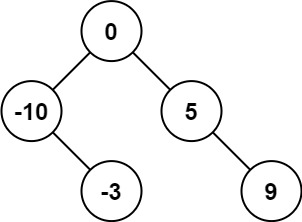# 108. Convert Sorted Array to Binary Search Tree￼

Given an integer array `nums` where the elements are sorted in ascending order, convert it to a height-balanced binary search tree.

height-balanced binary tree is a binary tree in which the depth of the two subtrees of every node never differs by more than one.

Example 1:

```Input: nums = [-10,-3,0,5,9]
Output: [0,-3,9,-10,null,5]
Explanation: [0,-10,5,null,-3,null,9] is also accepted:```

``````/**
* Definition for a binary tree node.
* function TreeNode(val, left, right) {
*     this.val = (val===undefined ? 0 : val)
*     this.left = (left===undefined ? null : left)
*     this.right = (right===undefined ? null : right)
* }
*/
/**
* @param {number[]} nums
* @return {TreeNode}
*/
var sortedArrayToBST = function(nums) {

return divide(0,nums.length-1)

function divide(start, end){

if(end < start) return null

let arrMid = Math.floor((start + end)/2);

let node = new TreeNode(nums[arrMid])

node.left = divide(start , arrMid-1)
node.right = divide(arrMid+1 , end)

return node

}

};``````